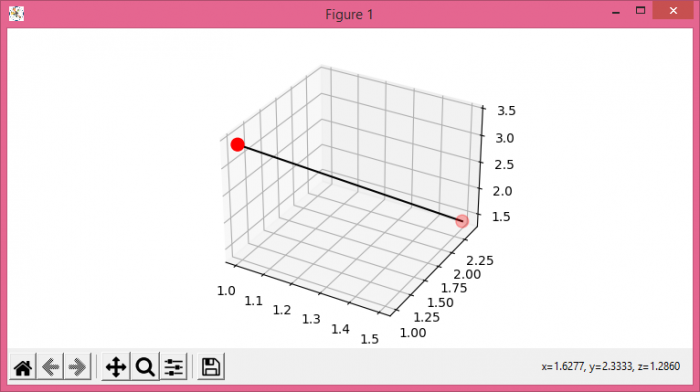# Connecting two points on a 3D scatter plot in Python and Matplotlib

MatplotlibPythonData Visualization

To connect two points on a 3D scatter plot, we can take the following steps

• Set the figure size and adjust the padding between and around the subplots.
• Create a new figure or activate an existing figure using figure() method.
• Add an axes to the current figure as a subplot arrangement.
• Create lists for x, y and z.
• Plot x, y and z data points using scatter() method
• To connect the points, use plot() method with x, y and z data points with black color line.
• To display the figure, use show() method.

## Example

from matplotlib import pyplot as plt
plt.rcParams["figure.figsize"] = [7.50, 3.50]
plt.rcParams["figure.autolayout"] = True
fig = plt.figure()
plt.show()